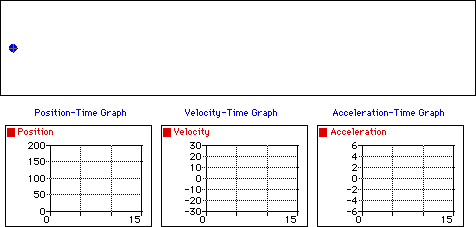## Monday, December 10, 2007

### MU120: Velocity, Speed & Acceleration

Having started Unit 11 "Movement" I have been struggling to get my head around velocity, and how it differs to speed and acceleration.  After having done some further research on the net I came across a web-site called The Physics Classroom which not only had an understandable lesson on "Speed and Velocity" but also some simple animations that clearly shows the difference.

Positive Velocity and Positive Acceleration

Observe that the object below moves in the positive direction with a changing velocity. An object which moves in the positive direction has a positive velocity. If the object is speeding up, then its acceleration vector is directed in the same direction as its motion (in this case, a positive acceleration). The "ticker tape" shows that each consecutive dot is not the same distance apart (i.e., a changing velocity). The position-time graph shows that the slope is changing (meaning a changing velocity) and positive (meaning a positive velocity). The velocity-time graph shows a line with a positive (upward) slope (meaning that there is a positive acceleration); the line is located in the positive region of the graph (corresponding to a positive velocity). The acceleration-time graph shows a horizontal line in the positive region of the graph (meaning a positive acceleration).